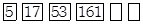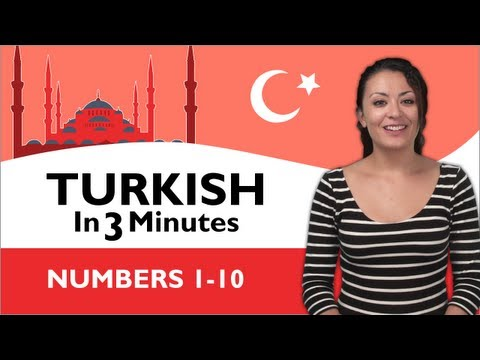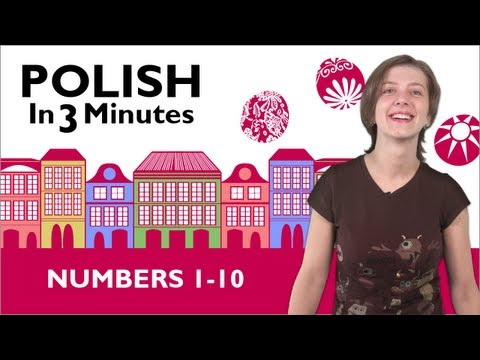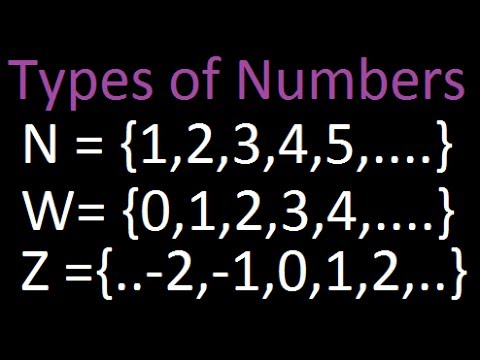Courses

Test: Numbers - 1

10 Questions MCQ Test Mathematics for Class 4: NCERT | Test: Numbers - 1

Description
Attempt Test: Numbers - 1 | 10 questions in 20 minutes | Mock test for Class 4 preparation | Free important questions MCQ to study Mathematics for Class 4: NCERT for Class 4 Exam | Download free PDF with solutions
QUESTION: 1

A and B are natural numbers. Value of A is twice of B.B is 254689 more than 654123. Find the successor of A.

Solution:

B is 254689 more than 654123

=> B = 654123 + 254689

=> B = 908812

A is twice of B

=> A = 2B

=> A = 2 * 908812

=> A = 1817624

The successor of a whole number is the number obtained by adding 1 to it

successor of A​ =  1817624 + 1 =  1817625

QUESTION: 2

Solution:
QUESTION: 3

Write the expanded form for numeral 7400546

Solution:
QUESTION: 4

Write the number, seventy five crore five lakh thirteen thousand two hundred nineteen, as per international system of numeration.

Solution:
QUESTION: 5

How many times does the place value of 8 in the numeral 2583219705 is greater than the face value of 8?

Solution:
QUESTION: 6

In the numeral 45695A221442, name the period that A occupies.

Solution:
QUESTION: 7

We can arrange the numbers in many specific way. Ascending and descending order of numbers are also a kind of arrangement of numbers, In descending order numbers are arranged from.

Solution:
QUESTION: 8

Which one of the following is not true.

Solution:

The predecessor of an even number is an odd number.

The successor of an even number is always an odd number. For example, after 26, 27 will come.

QUESTION: 9

Jack selects the number 542631 and finds its predecessor. If he increases the number by 2348795, by how much the predecessor will increase.

Solution:
QUESTION: 10

Complete the following number series.Solution:

485 and 1455Use Code STAYHOME200 and get INR 200 additional OFF Use Coupon Code

Track your progress, build streaks, highlight & save important lessons and more!

Similar ContentRelated tests